International
Tables for
Crystallography
Volume B
Reciprocal space
Edited by U. Shmueli

International Tables for Crystallography (2006). Vol. B. ch. 2.5, p. 325   | 1 | 2 |

## Section 2.5.7.7. Maximum entropy and likelihood

D. L. Dorsete*

#### 2.5.7.7. Maximum entropy and likelihood

| top | pdf |

Maximum entropy has been applied to electron crystallography in several ways. In the sense that images are optimized, the entropy term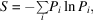where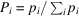and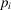is a pixel density, has been evaluated for various test electron-microscope images. For crystals, the true projected potential distribution function is thought to have the maximum value of S. If the phase contrast transfer function used to obtain a micrograph is unknown, test images (i.e. trial potential maps) can be calculated for different values of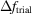. The value that corresponds to the maximum entropy would be near the true defocus. In this way, the actual objective lens transfer function can be found for a single image (Li, 1991) in addition to the other techniques suggested by this group.

Another use of the maximum-entropy concept is to guide the progress of a direct phase determination (Bricogne & Gilmore, 1990; Gilmore et al., 1990). Suppose that there is a small set H of known phases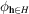(corresponding either to origin definition, or the Fourier transform of an electron micrograph, or both) with associated unitary structure-factor amplitudes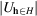. [The unitary structure factor is defined as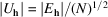.] As usual, the task is to expand into the unknown phase set K to solve the crystal structure. From Bayes' theorem, the procedure is based on an operation where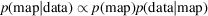. This means that the probability of successfully deriving a potential map, given diffraction data, is estimated. This so-called posterior probability is approximately proportional to the product of the probability of generating the map (known as the prior) and the probability of generating the data, given the map (known as the likelihood). The latter probability consults the observed data and can be used as a figure of merit.

Beginning with the basis set H, a trial map is generated from the limited number of phased structure factors. As discussed above, the map can be immediately improved by removing all negative density. The map can be improved further if its entropy is maximized using the equation given above for S. This produces the so-called maximum-entropy prior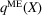.

So far, it has been assumed that all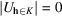. If large reflections from the K set are now added and their phase values are permuted, then a number of new maps can be generated and their entropies can be maximized as before. This creates a phasing tree' with many possible solutions; individual branch points can have further reflections added via permutations to produce further sub-branches, and so on. Obviously, some figure of merit is needed to prune' the tree, i.e. to find likely paths to a solution.

The desired figure of merit is the likelihood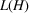. First a quantity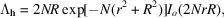where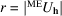(the calculated unitary structure factors) and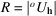(the observed unitary structure factors), is defined. From this one can calculate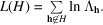The null hypothesis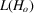can also be calculated from the above when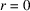, so that the likelihood gain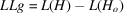ranks the nodes of the phasing tree in order of the best solutions.

Applications have been made to experimental electron-crystallographic data. A small-molecule structure starting with phases from an electron micrograph and extending to electron-diffraction resolution has been reported (Dong et al., 1992). Other experimental electron-diffraction data sets used in other direct phasing approaches (see above) also have been assigned phases by this technique (Gilmore, Shankland & Bricogne, 1993). These include intensities from diketopiperazine and basic copper chloride. An application of this procedure in protein structure analysis has been published by Gilmore et al. (1992) and Gilmore, Shankland & Fryer (1993). Starting with 15 Å phases, it was possible to extend phases for bacteriorhodopsin to the limits of the electron-diffraction pattern, apparently with greater accuracy than possible with the Sayre equation (see above).

### ReferencesBricogne, G. & Gilmore, C. J. (1990). A multisolution method of phase determination by combined maximization of entropy and likelihood. I. Theory, algorithms and strategy. Acta Cryst. A46, 284–297.Google ScholarDong, W., Baird, T., Fryer, J. R., Gilmore, C. J., MacNicol, D. D., Bricogne, G., Smith, D. J., O'Keefe, M. A. & Hovmöller, S. (1992). Electron microscopy at 1 Å resolution by entropy maximization and likelihood ranking. Nature (London), 355, 605–609.Google ScholarGilmore, C. J., Bricogne, G. & Bannister, C. (1990). A multisolution method of phase determination by combined maximization of entropy and likelihood. II. Application to small molecules. Acta Cryst. A46, 297–308.Google ScholarGilmore, C. J., Shankland, K. & Bricogne, G. (1993). Applications of the maximum entropy method to powder diffraction and electron crystallography. Proc. R. Soc. London Ser. A, 442, 97–111.Google ScholarGilmore, C. J., Shankland, K. & Fryer, J. R. (1992). The application of the maximum entropy method to electron microscopy data for purple membrane. Trans. Am. Crystallogr. Assoc. 28, 129–139.Google ScholarGilmore, C. J., Shankland, K. & Fryer, J. R. (1993). Phase extension in electron crystallography using the maximum entropy method and its application to two-dimensional purple membrane data from Halobacterium halobium. Ultramicroscopy, 49, 132–146.Google ScholarLi, F. H. (1991). Crystal structures from high-resolution electron microscopy. In Electron crystallography of organic molecules, edited by J. R. Fryer & D. L. Dorset, pp. 153–167. Dordrecht: Kluwer Academic Publishers.Google Scholar